Test: Linear Algebra

# Test: Linear Algebra - Electrical Engineering (EE)

Test Description

## 15 Questions MCQ Test GATE Electrical Engineering (EE) 2024 Mock Test Series - Test: Linear Algebra

Test: Linear Algebra for Electrical Engineering (EE) 2023 is part of GATE Electrical Engineering (EE) 2024 Mock Test Series preparation. The Test: Linear Algebra questions and answers have been prepared according to the Electrical Engineering (EE) exam syllabus.The Test: Linear Algebra MCQs are made for Electrical Engineering (EE) 2023 Exam. Find important definitions, questions, notes, meanings, examples, exercises, MCQs and online tests for Test: Linear Algebra below.
Solutions of Test: Linear Algebra questions in English are available as part of our GATE Electrical Engineering (EE) 2024 Mock Test Series for Electrical Engineering (EE) & Test: Linear Algebra solutions in Hindi for GATE Electrical Engineering (EE) 2024 Mock Test Series course. Download more important topics, notes, lectures and mock test series for Electrical Engineering (EE) Exam by signing up for free. Attempt Test: Linear Algebra | 15 questions in 45 minutes | Mock test for Electrical Engineering (EE) preparation | Free important questions MCQ to study GATE Electrical Engineering (EE) 2024 Mock Test Series for Electrical Engineering (EE) Exam | Download free PDF with solutions
 1 Crore+ students have signed up on EduRev. Have you?
Test: Linear Algebra - Question 1

### The rank of the following ( n + 1 ) x ( n + 1) matrix, where a is a real number is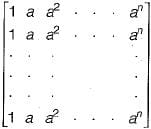Detailed Solution for Test: Linear Algebra - Question 1

All the rows of the given matrix is same. So the matrix has only one independent row.
Rank of the matrix = Number of independent rows of the matrix.
∴ Rank of given matrix = 1

Test: Linear Algebra - Question 2

### Let AX - B be a system of linear equations where A is an m x n matrix and b is a m x 1 column vector and X is an x 1 column vector of unknown. Which of the following is false?

Detailed Solution for Test: Linear Algebra - Question 2

Following are the possibilities for a system of linear equations:
(i) If matrix A and augmented matrix [AB] have same rank, then the system has solution otherwise there is no solution.
(ii) If matrix A and augmented matrix [AB] have same rank which is equal to the number of variables, then the system has unique solution and if B is zero vector then the system have only a trivial solution.
(iii) If matrix A and matrix [AB] have same rank which is less than the number of variables, then the system has infinite solution.
Therefore, option (c) is false because if m - n and B is non-zero vector, then it is not necessary that system has a unique solution, because m is the number of equations (quantity) and not the number of linearly independent equations (quality).

Test: Linear Algebra - Question 3

### The matrices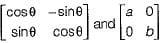commute under multiplication

Detailed Solution for Test: Linear Algebra - Question 3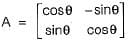and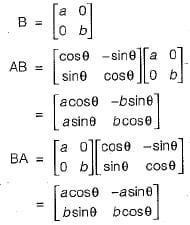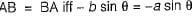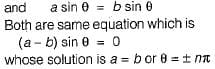Test: Linear Algebra - Question 4

Consider the following set of equations:
x + 2y = 5
4x + 8y = 12
3x + 6y + 3z = 15
This set

Detailed Solution for Test: Linear Algebra - Question 4

Set of equations is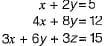Above set of equations can be written as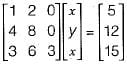Augmented matrix [AB] is given as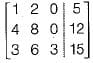Performing gauss-Elimination on the above matrix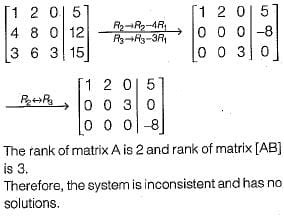Test: Linear Algebra - Question 5

An n x n array v is defined as follows: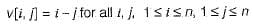The sum of the elements of the array v is

Detailed Solution for Test: Linear Algebra - Question 5

The matrix V can be defined as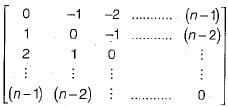So above is antisymmetric matrix and the sum of the elements of any antisymmetric matrix is 0.
OR
Alternate Method: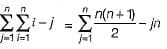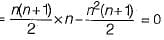Test: Linear Algebra - Question 6

Consider the following statements:
S1: The sum of two singular n x n matrices may be non-singular
S2: The sum of two n x n non-singular matrices may be singular
Which of the following statements is correct?

Detailed Solution for Test: Linear Algebra - Question 6

S1 is true
Consider two singular matrices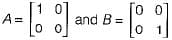Sum of A and B is given as
However (A + B) is a non-singuiar matrix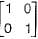So, S1 is true.
Now, consider two non-singuiar matrices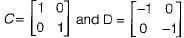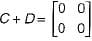However (C + D) is a singular matrix. So, S2 is also true.
Therefore, both S1 and S2 are true.

Test: Linear Algebra - Question 7

Let A, B, C, D be n x n matrices, each with non-zero determinant, If ABCD = I, then B-1 is

Detailed Solution for Test: Linear Algebra - Question 7

A, B, C, D is n x n matrix.
Given, ABCD = I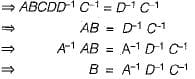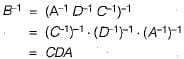Test: Linear Algebra - Question 8

What values of x, y and z satisfy the following system of linear equations?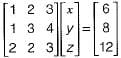Detailed Solution for Test: Linear Algebra - Question 8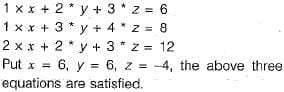Test: Linear Algebra - Question 9

How many solutions does the following system of linear equations have?
- x + 5y = - 1
x - y = 2
x + 3y = 3

Detailed Solution for Test: Linear Algebra - Question 9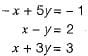The augmented matrix is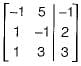Using gauss-efimination on above matrix we get,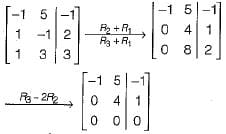Rank [A | B] - 2 (number of non zero rows in [A | B])
Rank [A ] = 2 (number of non zero row s in [A ])
Rank [A | B] = Rank [A] = 2 = number of variables
∴ Unique solution exists.

Test: Linear Algebra - Question 10

Consider the following system of equations in three real variables x1, x2 and x3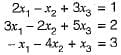This system of equations has

Detailed Solution for Test: Linear Algebra - Question 10

The augmented matrix for the given system is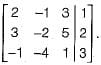Using gauss -elimination method on above matrix we get,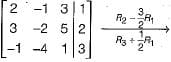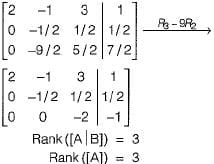Since Rank ([A | B]) = Rank ([A]) = number of variables, the system has unique solution.

Test: Linear Algebra - Question 11

What are the eigenvalues of the following 2 x 2 matrix?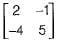Detailed Solution for Test: Linear Algebra - Question 11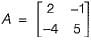The characteristic equation of this matrix is given by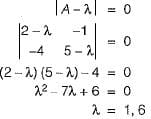∴ The eigen values of A are 1 and 6.

Test: Linear Algebra - Question 12

F is an n x n real matrix, b is an n x 1 real vector. Suppose there are two n x 1 vectors, u and v such that u ≠ v and Fu = b, Fv = b.
Which one of the following statements is false?

Detailed Solution for Test: Linear Algebra - Question 12

Given that Fu= b and Fv= b
If F is non singular, then it has a unique inverse.
Now, u = F-1 b and v = F-1 b
Since F-1 is unique u = v but it is given that u ≠ v .
This is a contradiction. So F must be singular.
This means that
(a) Determinate of F is zero is true. Also
(b) There are infinite number of solution to Fx = b is true since |F| = 0
(c) There is an X ≠ 0 such that FX = 0 is also true, since X has infinite number of solutions, including the X = 0 solution.
(d) F must have 2 identical rows is false, since a determinant may become zero, even if two identical columns are present. It is not necessary that 2 identical rows must be present for |F| to become zero.

Test: Linear Algebra - Question 13

What are the eigenvalues of the matrix P given below: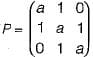Detailed Solution for Test: Linear Algebra - Question 13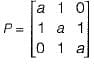Since P is a square matrix
Sum (eigen values) = Trace (P)
= a + a + a = 3a
Product of eigen values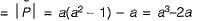Only choice (a) has sum of eigen values = 3a and product of eigen values = a3 - 2a.

Test: Linear Algebra - Question 14

If the rank of a (5 x 6) matrix Q is 4, then which one of the following statements is correct?

Detailed Solution for Test: Linear Algebra - Question 14

If rank of (5 x 6) matrix is 4, then surely it must have exactly 4 linearly independent rows as will as 4 linearly in dependent columns.

Test: Linear Algebra - Question 15

The trace and determinant of a 2 x 2 matrix are known to be -2 and -35 respectively. It eigenvalues are

Detailed Solution for Test: Linear Algebra - Question 15

Trace = Sum of principal diagonal element.

## GATE Electrical Engineering (EE) 2024 Mock Test Series

23 docs|285 tests
Information about Test: Linear Algebra Page
In this test you can find the Exam questions for Test: Linear Algebra solved & explained in the simplest way possible. Besides giving Questions and answers for Test: Linear Algebra, EduRev gives you an ample number of Online tests for practice

## GATE Electrical Engineering (EE) 2024 Mock Test Series

23 docs|285 tests